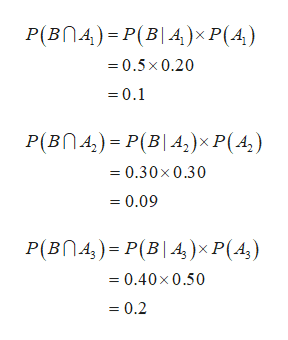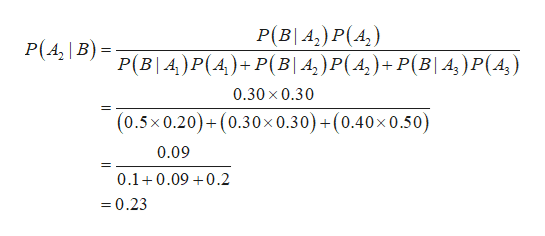# The prior probabilities for eventsA1, A2, and A3areP(A1) = 0.20,P(A2) = 0.30,andP(A3) = 0.50.The conditional probabilities of event B givenA1,A2,andA3areP(B | A1) = 0.50,P(B | A2) = 0.30,andP(B | A3) = 0.40.(Assume thatA1, A2, and A3are mutually exclusive events whose union is the entire sample space.)(a)ComputeP(B ∩ A1), P(B ∩ A2), and P(B ∩ A3).P(B ∩ A1)=P(B ∩ A2)=P(B ∩ A3)=(b)Apply Bayes' theorem,P(Ai | B) = P(Ai)P(B | Ai)P(A1)P(B | A1) + P(A2)P(B | A2) +    + P(An)P(B | An),to compute the posterior probabilityP(A2 | B).(Round your answer to two decimal places.) (c)Use the tabular approach to applying Bayes' theorem to computeP(A1 | B),P(A2 | B),andP(A3 | B).(Round your answers to two decimal places.)EventsP(Ai)P(B | Ai)P(Ai ∩ B)P(Ai | B)A10.200.50  A20.300.30  A30.500.40   1.00  1.00

Question
The prior probabilities for events
A1, A2, and A3
are
P(A1) = 0.20,
P(A2) = 0.30,
and
P(A3) = 0.50.
The conditional probabilities of event B given
A1,
A2,
and
A3
are
P(B | A1) = 0.50,
P(B | A2) = 0.30,
and
P(B | A3) = 0.40.
(Assume that
A1, A2, and A3
are mutually exclusive events whose union is the entire sample space.)
(a)
Compute
P(B ∩ A1), P(B ∩ A2), and P(B ∩ A3).
P(B ∩ A1)
=
P(B ∩ A2)
=
P(B ∩ A3)
=
(b)
Apply Bayes' theorem,
P(Ai | B) =
 P(Ai)P(B | Ai) P(A1)P(B | A1) + P(A2)P(B | A2) +    + P(An)P(B | An)
,
to compute the posterior probability
P(A2 | B).

(c)
Use the tabular approach to applying Bayes' theorem to compute
P(A1 | B),
P(A2 | B),
and
P(A3 | B).
Events
P(Ai)
P(B | Ai)
P(Ai ∩ B)
P(Ai | B)
A1
0.20 0.50
A2
0.30 0.30
A3
0.50 0.40
1.00     1.00
check_circle

Step 1

(a)

From the given information, P(A1)=0.20, P(A2)=0.30, P(A3)=0.50, P(B|A1)=0.5, P(B|A2)=0.30 and P(B|A3)=0.40.help_outlineImage TranscriptioncloseP(Bn4) P(B 4)x P(4) =0.5x 0.20 0.1 P(Bn4) P(B|A,) x P(A, ) 0.30x 0.30 = 0.09 P(Bn4,) P(B|A,)x P(A,) 0.40 x 0.50 0.2 fullscreen
Step 2

(b)

The value of P(A2|B) is 0.23 and it is calculated...help_outlineImage TranscriptioncloseP(B A,) P(4) P(B A) P(A)+P(B| A,) P(A)+ P(B| A,)P(A,) P(A B) 0.30 x 0.30 +(0.30x 0.30)+(0.40x 0.50) (0.5x0.20)+ 0.09 0.1 0.09 0.2 =0.23 fullscreen

### Want to see the full answer?

See Solution

#### Want to see this answer and more?

Solutions are written by subject experts who are available 24/7. Questions are typically answered within 1 hour.*

See Solution
*Response times may vary by subject and question.
Tagged in

### Other